Origin Of Quantum Mechanics NAT Level – 1

# Origin Of Quantum Mechanics NAT Level – 1

Test Description

## 10 Questions MCQ Test Topic wise Tests for IIT JAM Physics | Origin Of Quantum Mechanics NAT Level – 1

Origin Of Quantum Mechanics NAT Level – 1 for IIT JAM 2023 is part of Topic wise Tests for IIT JAM Physics preparation. The Origin Of Quantum Mechanics NAT Level – 1 questions and answers have been prepared according to the IIT JAM exam syllabus.The Origin Of Quantum Mechanics NAT Level – 1 MCQs are made for IIT JAM 2023 Exam. Find important definitions, questions, notes, meanings, examples, exercises, MCQs and online tests for Origin Of Quantum Mechanics NAT Level – 1 below.
Solutions of Origin Of Quantum Mechanics NAT Level – 1 questions in English are available as part of our Topic wise Tests for IIT JAM Physics for IIT JAM & Origin Of Quantum Mechanics NAT Level – 1 solutions in Hindi for Topic wise Tests for IIT JAM Physics course. Download more important topics, notes, lectures and mock test series for IIT JAM Exam by signing up for free. Attempt Origin Of Quantum Mechanics NAT Level – 1 | 10 questions in 30 minutes | Mock test for IIT JAM preparation | Free important questions MCQ to study Topic wise Tests for IIT JAM Physics for IIT JAM Exam | Download free PDF with solutions
 1 Crore+ students have signed up on EduRev. Have you?
*Answer can only contain numeric values
Origin Of Quantum Mechanics NAT Level – 1 - Question 1

### An X-ray tube operating at 50kV converts 1%  energy in the form of X-ray. If the amount of heat produced is 495 watts, then the number of electrons colliding with the target per second is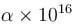. Find the value of α.

Detailed Solution for Origin Of Quantum Mechanics NAT Level – 1 - Question 1

Number of electrons colliding with the target per second is :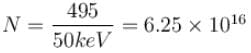*Answer can only contain numeric values
Origin Of Quantum Mechanics NAT Level – 1 - Question 2

### In a hydrogen atom following Bohr's postulates, the product of linear momentum and angular momentum is proportional to (n)x where n is the orbit number. Then x is

Detailed Solution for Origin Of Quantum Mechanics NAT Level – 1 - Question 2

Linear momentum ⇒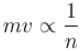Angular momentum ⇒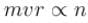∴  Product of linear momentum and angular momentum ∝ n0.

*Answer can only contain numeric values
Origin Of Quantum Mechanics NAT Level – 1 - Question 3

### Work function for aluminum metal is  4.125 eV.  For photo electric effect from aluminum metal, the cut off wavelength (in nm) will be.

Detailed Solution for Origin Of Quantum Mechanics NAT Level – 1 - Question 3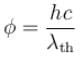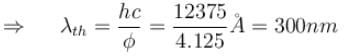*Answer can only contain numeric values
Origin Of Quantum Mechanics NAT Level – 1 - Question 4

If the short wavelength limit of the continuous spectrum coming out of a coolidge tube is 10Å, then the de-Broglie wavelength (in Å)of the electrons reaching the largest metal in the coolidge tube is approximately.

Detailed Solution for Origin Of Quantum Mechanics NAT Level – 1 - Question 4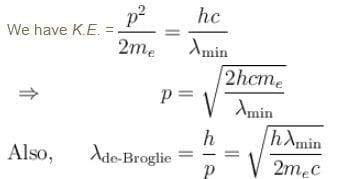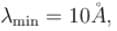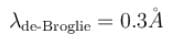*Answer can only contain numeric values
Origin Of Quantum Mechanics NAT Level – 1 - Question 5

Yellow light of 557 nm wavelength is incident on a cesium surface. It is found that no photo-electron flow in the circuit when the cathode anode voltage drops below 0.25V. Then the threshold wavelength (in nm) for photoelectric effect from cesium is.

Detailed Solution for Origin Of Quantum Mechanics NAT Level – 1 - Question 5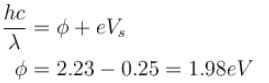⇒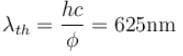*Answer can only contain numeric values
Origin Of Quantum Mechanics NAT Level – 1 - Question 6

Light of wavelength 5000Å falls on a sensitive plate with photoelectric work function of 1.9 eV. The kinetic energy (in eV) of the emitted photo-electron will be.

Detailed Solution for Origin Of Quantum Mechanics NAT Level – 1 - Question 6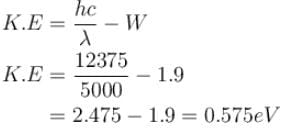*Answer can only contain numeric values
Origin Of Quantum Mechanics NAT Level – 1 - Question 7

The ratio of de-Broglie wavelength of a proton and an α-particle accelerated through the same potential difference is:

Detailed Solution for Origin Of Quantum Mechanics NAT Level – 1 - Question 7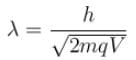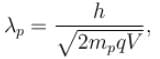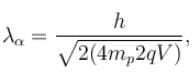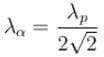⇒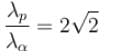*Answer can only contain numeric values
Origin Of Quantum Mechanics NAT Level – 1 - Question 8

When light of intensity 1 W/m2 and wavelength 5 × 10–7 m is incident on a surface, it is completely absorbed by the surface. If 100 photons emit one electron and area of the surface is 1 cm2, then the photoelectric current (in µA) will be.

Detailed Solution for Origin Of Quantum Mechanics NAT Level – 1 - Question 8

Number of photon/second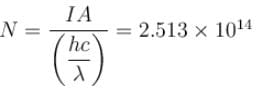Number of photon electron emitted/second by 1 photon = N/100
Photo electric current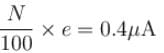*Answer can only contain numeric values
Origin Of Quantum Mechanics NAT Level – 1 - Question 9

The wavelength of Ln line in X-rays spectrum of 78Pt is 1.32 Å. The wavelength of Ln line in X-rays spectrum of another unknown element is 4.17Å. If screening constant for La line is 7.4, then atomic number of the unknown element is :

Detailed Solution for Origin Of Quantum Mechanics NAT Level – 1 - Question 9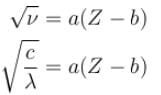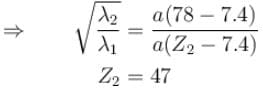*Answer can only contain numeric values
Origin Of Quantum Mechanics NAT Level – 1 - Question 10

The intensity of an X-ray beam reduces to 36.8% of its initial intensity after traversing a gold film of thickness 5 × 10–3 m. Its absorption coefficient (in m–1) is :

Detailed Solution for Origin Of Quantum Mechanics NAT Level – 1 - Question 10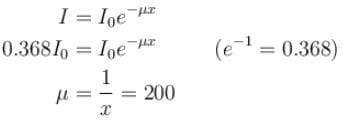## Topic wise Tests for IIT JAM Physics

217 tests
 Use Code STAYHOME200 and get INR 200 additional OFF Use Coupon Code
Information about Origin Of Quantum Mechanics NAT Level – 1 Page
In this test you can find the Exam questions for Origin Of Quantum Mechanics NAT Level – 1 solved & explained in the simplest way possible. Besides giving Questions and answers for Origin Of Quantum Mechanics NAT Level – 1, EduRev gives you an ample number of Online tests for practice

217 tests